Anúncio

# CAPACITORS.pptx

26 de Mar de 2023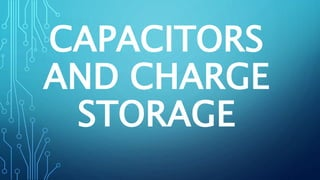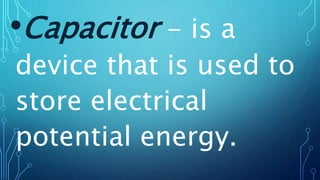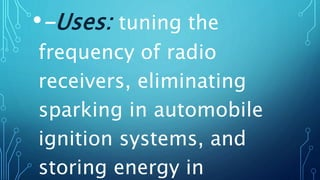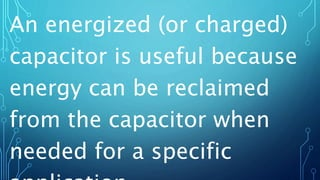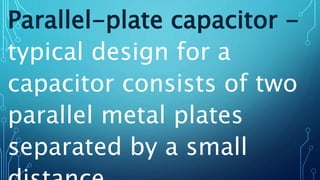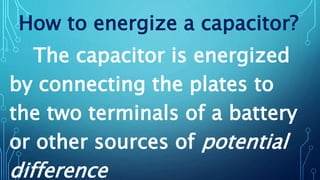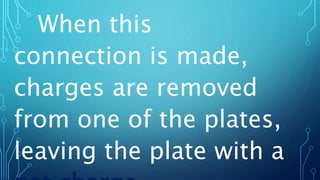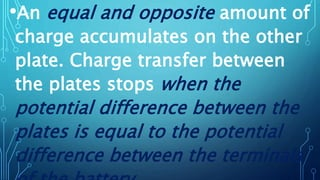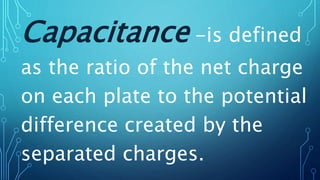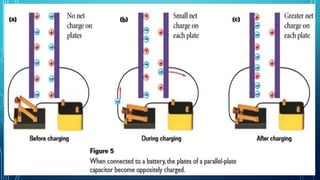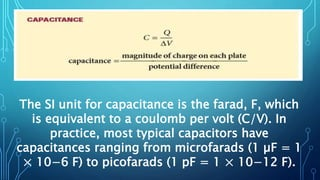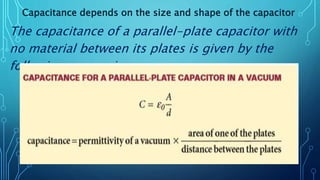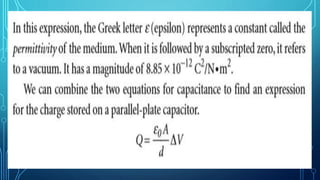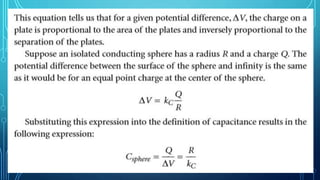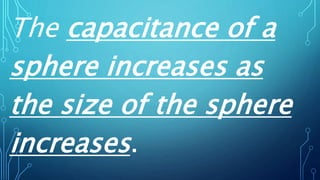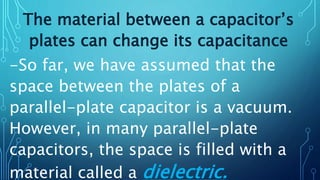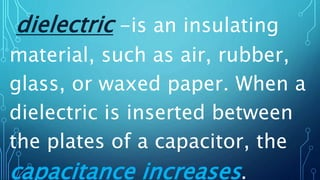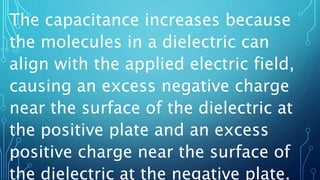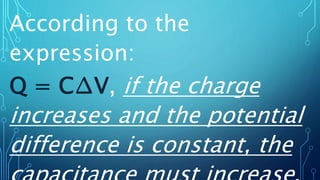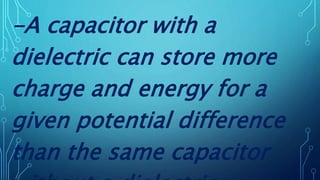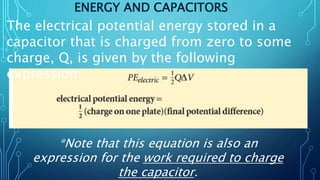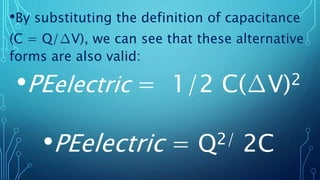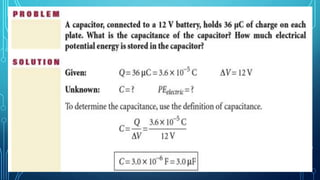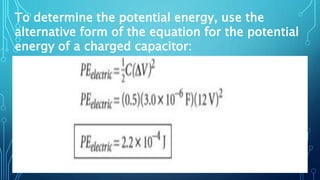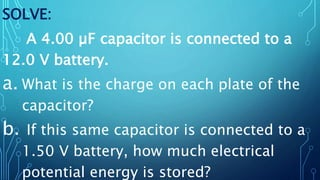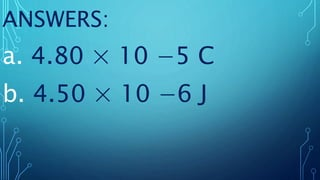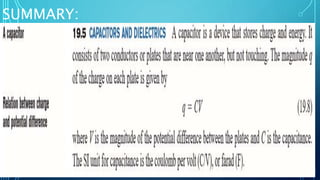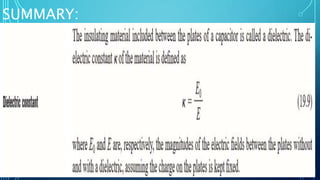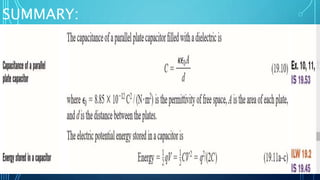1 de 29
Anúncio

### CAPACITORS.pptx

1. CAPACITORS AND CHARGE STORAGE
2. •Capacitor - is a device that is used to store electrical potential energy.
3. •-Uses: tuning the frequency of radio receivers, eliminating sparking in automobile ignition systems, and storing energy in
4. An energized (or charged) capacitor is useful because energy can be reclaimed from the capacitor when needed for a specific
5. Parallel-plate capacitor - typical design for a capacitor consists of two parallel metal plates separated by a small
6. How to energize a capacitor? The capacitor is energized by connecting the plates to the two terminals of a battery or other sources of potential difference
7. When this connection is made, charges are removed from one of the plates, leaving the plate with a
8. •An equal and opposite amount of charge accumulates on the other plate. Charge transfer between the plates stops when the potential difference between the plates is equal to the potential difference between the terminals
9. Capacitance -is defined as the ratio of the net charge on each plate to the potential difference created by the separated charges.
10. The SI unit for capacitance is the farad, F, which is equivalent to a coulomb per volt (C/V). In practice, most typical capacitors have capacitances ranging from microfarads (1 µF = 1 × 10−6 F) to picofarads (1 pF = 1 × 10−12 F).
11. Capacitance depends on the size and shape of the capacitor The capacitance of a parallel-plate capacitor with no material between its plates is given by the following expression:
12. The capacitance of a sphere increases as the size of the sphere increases.
13. The material between a capacitor’s plates can change its capacitance -So far, we have assumed that the space between the plates of a parallel-plate capacitor is a vacuum. However, in many parallel-plate capacitors, the space is filled with a material called a dielectric.
14. dielectric -is an insulating material, such as air, rubber, glass, or waxed paper. When a dielectric is inserted between the plates of a capacitor, the capacitance increases.
15. The capacitance increases because the molecules in a dielectric can align with the applied electric field, causing an excess negative charge near the surface of the dielectric at the positive plate and an excess positive charge near the surface of the dielectric at the negative plate.
16. According to the expression: Q = C∆V, if the charge increases and the potential difference is constant, the
17. -A capacitor with a dielectric can store more charge and energy for a given potential difference than the same capacitor
18. ENERGY AND CAPACITORS The electrical potential energy stored in a capacitor that is charged from zero to some charge, Q, is given by the following expression: *Note that this equation is also an expression for the work required to charge the capacitor.
19. •By substituting the definition of capacitance (C = Q/∆V), we can see that these alternative forms are also valid: •PEelectric = 1/2 C(∆V)2 •PEelectric = Q2/ 2C
20. To determine the potential energy, use the alternative form of the equation for the potential energy of a charged capacitor:
21. SOLVE: A 4.00 µF capacitor is connected to a 12.0 V battery. a. What is the charge on each plate of the capacitor? b. If this same capacitor is connected to a 1.50 V battery, how much electrical potential energy is stored?
22. ANSWERS: a. 4.80 × 10 −5 C b. 4.50 × 10 −6 J
23. SUMMARY:
24. SUMMARY:
25. SUMMARY:
Anúncio